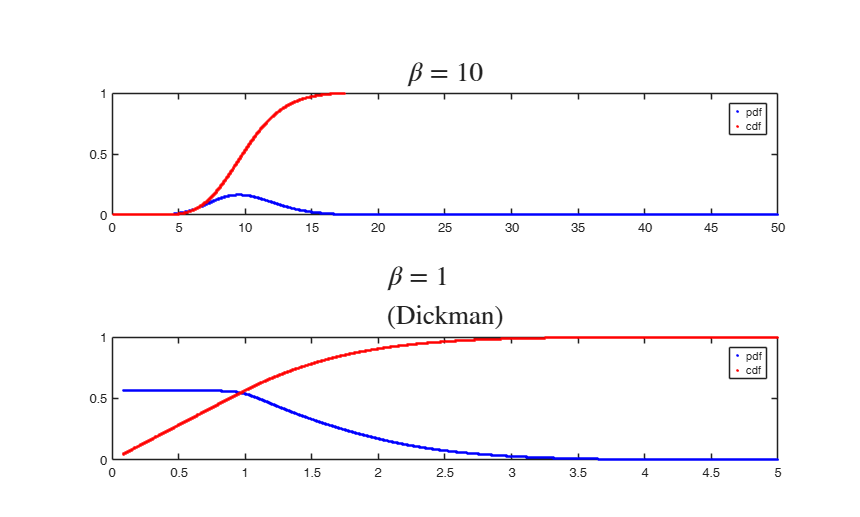# vervaatxdf

vervaatxdf returns the pdf and cdf of a Vervaat perpetuity.

## Syntax

• f =vervaatxdf(betav)example
• f =vervaatxdf(betav,nx)example
• f =vervaatxdf(betav,nx,pascalM)example
• [f , F ]=vervaatxdf(___)example
• [f , F , x]=vervaatxdf(___)example

## Description

The pdf and cdf of a Vervaat perpetuity with parameter $\beta \in (0,+\infty)$, estimated following Barabesi and Pratelli (2019).

 f =vervaatxdf(betav) A Dickman value (Vervaat perpetuity with $\beta = 1$).

 f =vervaatxdf(betav, nx) nx Dickman values.

 f =vervaatxdf(betav, nx, pascalM) Use of pascal matrix in computing the Dickman values.

 [f , F ] =vervaatxdf(___) Comparison of Vervaat perpetuities of different parameter values.

 [f , F , x] =vervaatxdf(___) A rough time test on the method, for different sample sizes and beta values.

## Examples

expand all

### A Dickman value (Vervaat perpetuity with $\beta = 1$).

clear all; close all;
[f F x] = vervaatxdf(1);

### nx Dickman values.

clear all; close all;
nx = 100;
[f F x] = vervaatxdf(1,nx);

### Use of pascal matrix in computing the Dickman values.

clear all; close all;
[f F x] = vervaatxdf(1,100, pascal(101));

###Comparison of Vervaat perpetuities of different parameter values.

Parameters: $\beta = 1$ and $\beta = 10$.

clear all; close all;
betav10 = 10;
betav01 = 1;
N = 10000;
[f10 F10 x10] = vervaatxdf(betav10,N);
[f01 F01 x01] = vervaatxdf(betav01,N);
%     % remove inf and nan
%     maskinf = not(isinf(F10));
%     masknan = not(isnan(F10));
%     x10 = x10(mask); F10 = F10(mask); f10 = f10(mask);
%     maskinf = not(isinf(F01));
%     masknan = not(isnan(F01));
%     x01 = x01(mask); F01 = F01(mask); f01 = f01(mask);
figure;
h1=subplot(2,1,1);
plot(x10,f10,'.b','LineWidth',2); ylim([0,1]);
hold on
plot(x10,F10,'.r','LineWidth',2); ylim([0,1]);
legend('pdf','cdf');
h2=subplot(2,1,2);
plot(x01,f01,'.b','LineWidth',2); ylim([0,1]);
hold on
plot(x01,F01,'.r','LineWidth',2); ylim([0,1]);
legend('pdf','cdf');
title(h1,'$$\beta = 10$$' ,'Fontsize',20,'interpreter','latex');
title(h2,'$$\beta = 1$$ (Dickman)' ,'Fontsize',20,'interpreter','latex');### A rough time test on the method, for different sample sizes and beta values.

clear all;
close all;
betav = 1;
n = 100;
nmax    = 500;
betamax = 10;
rng(123);
N     = randi(nmax,n,1);
betav = randi(betamax,n,1);
ti = tic;
for i=1:n;
for j=1:N(i)
y1(j) = vervaatxdf(betav(i));
end
end
tf = toc(ti);
tip = tic;
pascalM = pascal(101);
for i=1:n;
for j=1:N(i)
y1p(j) = vervaatxdf(betav(i),1,pascalM);
end
end
tfp = toc(tip);
disp(['Barabesi-Pratelli: etime = ' num2str(tf)]);
disp(['Barabesi-Pratelli - Pascal run only once: etime = ' num2str(tfp)]);

## Input Arguments

### betav — Distribution parameter value. Positive integer.

The parameter of the Vervaat family. Default is betav = 1.

Data Types: single| double

### nx — Number of evaluation points. Positive integer.

The nx evaluation points are chosen randomly from a uniform in a range covering the support of the distribution, which depends on the parameter beta. Default is n = 1.

Example: n=1000. 

Data Types: Scalar.

### pascalM — A precomputed Pascal matrix, used to speed up vervaatxdf in simulations. The order Pascal of the matrix must be 101: this is required by the internal function structure.

The matrix is obtained with pascalM = pascal(101). The option can be used in simulations where vervaatxdf is called many times, so that to avoid the recalculation of the pascal matrix. Internally, the pascal matrix is used to avoid the reiterated computation of the binomial coefficient, which would be extremely time consuming.

Example: pascalM = pascal(101). 

Data Types: Scalar.

## Output Arguments

### f —The pdf of the Vervaat perpetuity value. Array

The probability density function estimated on nx random evaluation points.

Data Types - Double.

### F —The cdf of the Vervaat perpetuity value. Array

The cumulative density function corresponding to f.

Data Types - Double.

### x —Random evaluation points. Array

The nx evaluation points extracted from a uniform in the support of the distribution.

Data Types - Double.

A perpetuity is a random variable of the form:

$Y = W_1 + W_1 \cdot W_2 + W_1 \cdot W_2 \cdot W_3 + \ldots$

where the $W_i$ are an independent, identically distributed (iid) sequence of random variables. If each $W_i$ has the same distribution, say $W_i \sim W$, then $Y \sim W(1 + Y)$ for $Y$ and $W$ independent.

We are interested in this distribution because the running time of the Quickselect algorithm of Hoare, for finding the order statistics in a numerical array, approaches asymptotically a particular perpetuity, called Dickman distribution, with $W \sim Unif([0,1])$. Unfortunately such distribution has no closed form.

The Dickman distribution can be also seen as a special case of Vervaat perpetuity, which is such that $W_i \sim U^{1/\beta}$ for some $\beta \in (0,\infty)$ for $U \sim Unif([0,1])$. In other words, the Dickman distributon is a Vervaat perpetutiy with $\beta = 1$.

A generalization of the perpetuity takes the form

$Y = \sum_{n=0}^{\infty}A_n \prod_{i}^{n} W_i$

with $A_n$ not necessarily equal to $1$, which is known as Takacs distribution.

Barabesi, L. and Pratelli, L. (2019), On the properties of a Takacs distribution, "Statistics and Probability Letters", Vol. 148, pp. 66-73.

Cloud, K. and Huber, M. (2018), Fast Perfect Simulation of Vervaat Perpetuities, "Journal of Complexity", Vol. 42, pp. 19-30.

Devroye, L. (2001), Simulating perpetuities, "Methodology And Computing In Applied Probability", Vol. 3, Num. 1, pp. 97-115.

Fill, J. A. and Huber, M. (2010), Perfect simulation of Vervaat perpetuities, "Electronic Journal of Probability", Vol. 15, pp. 96-109.

Devroye, L. and Fawzi, O. (2010), Simulating the Dickman distribution, "Statistics and Probability Letters", Vol. 80, pp. 242-247.

Blanchet, J. H. and Sigman, K. (2011), On exact sampling of stochastic perpetuities, "Journal of Applied Probability", Vol. 48A, pp. 165-182.

Takacs, L. (1955), On stochastic processes connected with certain physical recording apparatuses. "Acta Mathematica Academiae Scientificarum Hungarica", Vol. 6, pp 363-379.